Courses

# Chemistry Test 4 - Solution, D And F Block Element, Metallurgy, Alcohol, Phenol And Ether

## 25 Questions MCQ Test Mock Test Series for JEE Main & Advanced 2021 | Chemistry Test 4 - Solution, D And F Block Element, Metallurgy, Alcohol, Phenol And Ether

Description
This mock test of Chemistry Test 4 - Solution, D And F Block Element, Metallurgy, Alcohol, Phenol And Ether for JEE helps you for every JEE entrance exam. This contains 25 Multiple Choice Questions for JEE Chemistry Test 4 - Solution, D And F Block Element, Metallurgy, Alcohol, Phenol And Ether (mcq) to study with solutions a complete question bank. The solved questions answers in this Chemistry Test 4 - Solution, D And F Block Element, Metallurgy, Alcohol, Phenol And Ether quiz give you a good mix of easy questions and tough questions. JEE students definitely take this Chemistry Test 4 - Solution, D And F Block Element, Metallurgy, Alcohol, Phenol And Ether exercise for a better result in the exam. You can find other Chemistry Test 4 - Solution, D And F Block Element, Metallurgy, Alcohol, Phenol And Ether extra questions, long questions & short questions for JEE on EduRev as well by searching above.
QUESTION: 1

Solution:
QUESTION: 2

Solution:
QUESTION: 3

### Among the following, which is least acidic?

Solution:
QUESTION: 4
Which compound is known as oil of winter green ?
Solution:
QUESTION: 5
When phenol is treated with excess bromine water
Solution:
QUESTION: 6
n-Propyl alcohol and iso-propyl alcohol can be chemically distinguished by which reagent
Solution:
QUESTION: 7
In the reaction of phenol with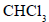and aqueous NaOH at (343 K), the electrophile attacking the ring is
Solution:
QUESTION: 8
Which of the following on oxidation gives a ketone ?
Solution:
QUESTION: 9
Dow’s reaction involves
Solution:
QUESTION: 10
The compound which gives the most stable carbonium ion on dehydration is
Solution:
QUESTION: 11
The compound obtained by the reaction of ethene with diborane followed by hydrolysis with alkaline hydrogen peroxide is
Solution:
QUESTION: 12
When benzenediazonium chloride is treated with water, the compound formed is
Solution:
QUESTION: 13
What amount of bromine will be required to convert 2g of phenol into 2,4, 6-tribromophenol?
Solution:
From the reaction we know that,

Phenol +  3 Br2  --------> 2,4,6-tribromophenol

For 3 Br2 = 3 * (2*80) = 480

Amount of bromine needed  ,

(480*2)/94 = 10.22

((94 is mass of phenol ))

QUESTION: 14

The reaction of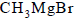with acetone and hydrolysis of the resulting product gives

Solution:
QUESTION: 15
Identify X in the following sequence of reaction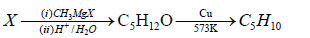Solution:
QUESTION: 16
Which one of the following pairs will not form an ideal solution ?
Solution:
QUESTION: 17
Which of the following pairs of solutions is isotonic ?
Solution:
QUESTION: 18
At 298K, highest osmotic pressure is exhibited by a 0.1M solution of
Solution:
QUESTION: 19
The van’t Hoff factor for a 0.1 M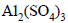solution is 4.20. The degree of dissociation is
Solution:
QUESTION: 20
The molal boiling-point constant for water is 0.513 K/m When 0.1 mole of sugar is dissolved in 200.0 g of water, the solution boils under a pressure of 1.0 atm at
Solution:
*Answer can only contain numeric values
QUESTION: 21

17.4 % (mass / volume) K2SO4 solution at 27°C is isotonic to 5.85% (mass / volume) NaCl solution at 27°C. If NaCl is 100% ionised, what is % ionisation of K2SO4 in aq. solution?

Solution: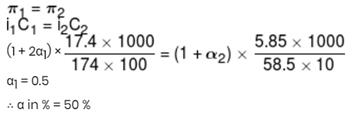*Answer can only contain numeric values
QUESTION: 22

Among the following ore how many example of carbonate ore:
lime stone, malachite, dolomite, Horn silver, Flourspar, Calamine, Cuprite.

Solution:
*Answer can only contain numeric values
QUESTION: 23

Identify the element for given which have the most negative electron gain enthalpy. Write atomic number of that element as answer :-
P ,  S,  Cl,  F,  Br

Solution:
*Answer can only contain numeric values
QUESTION: 24

Element 'X' has maximum electron affinity in periodic table then find the number of elements which have low first ionisation energy as compared to element 'X' ?
F, Na, S, Si, P, Ar

Solution:
*Answer can only contain numeric values
QUESTION: 25

If number of low spin complexes are 'X' then find the value of 1/x.
[Co(NH3)6]+3, [CoF6]–3, [Fe(CN)6]–4, [Fe(H2O)6]+2, [Cr(gly)3]0, [CoBr2Cl2]–2

Solution: